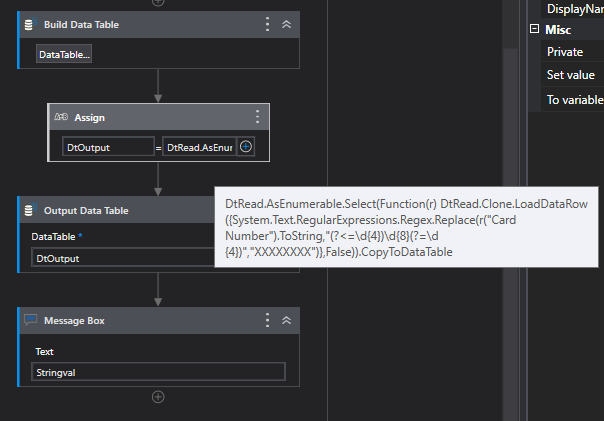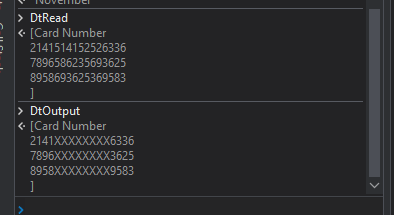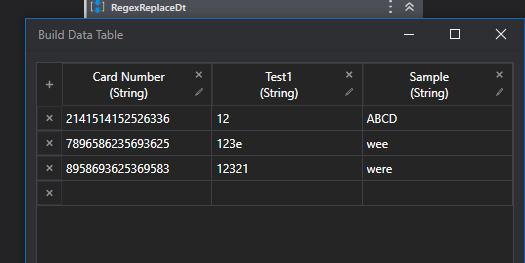# Mask card number column in excel

Hi,

I have more than 10000 rows, where I want to mask the card number column like:-

1st 4 number +“XXXXXXXX”(8 time X) + Last 4 number.

You can try with Regex Expression

``````System.Text.RegularExpressions.Regex.Replace(YourString,"(?<=\d{4})\d{8}(?=\d{4})","XXXXXXXX").Tostring
``````

Regards
Gokul

Thanks,

Actually as there are more than 10k rows. I want to avoid for each loop.

Any linq is there or VBA code?

``````DtRead.AsEnumerable.Select(Function(r) DtRead.Clone.LoadDataRow({System.Text.RegularExpressions.Regex.Replace(r("Card Number").ToString,"(?<=\d{4})\d{8}(?=\d{4})","XXXXXXXX")},False)).CopyToDataTable
``````

Check out the XAML file

RegexReplaceDt.xaml (7.1 KB)Regards
Gokul

Hi,

This works perfectly. But it removes other columns.

Can you share the column Headers?

Card Number, Customer Name, Outstanding, Out2, Limit, Cif, Sufix, Account Number

Here is the sample Input ScreenshotCheck out this expression i have added the additional columns `r("Column name")`

``````DtRead.AsEnumerable.Select(Function(r) DtRead.Clone.LoadDataRow({System.Text.RegularExpressions.Regex.Replace(r("Card Number").ToString,"(?<=\d{4})\d{8}(?=\d{4})","XXXXXXXX"),r("Test1"),r("Sample")},False)).CopyToDataTable
``````

Try with this

``````DtRead.AsEnumerable.Select(Function(r) DtRead.Clone.LoadDataRow({System.Text.RegularExpressions.Regex.Replace(r("Card Number").ToString,"(?<=\d{4})\d{8}(?=\d{4})","XXXXXXXX"),r("Customer Name"),r("Outstanding"),r("Out2"),r("Limit"),r("Cif"),r("Sufix"),r("Account Number")},False)).CopyToDataTable
``````

Regards
Gokul

2 Likes

This topic was automatically closed 3 days after the last reply. New replies are no longer allowed.